## Python随笔_孱弱Lynch的博客-程序员宅基地

6.3 实例9 基本统计值计算
6.6 实例10 文本词频统计

Python社区
https://pypi.org/

print()
print()函数默认在输出最后增加一个’\n’

### 字符串操作

#### 字符串切片

<字符串>[M:N] M缺省表示至开头，N缺省表示至结尾
<字符串>[M:N:K] 根据步长K对字符串进行切片

>>> "〇一二三四五六七八九十"[:3]
"〇一二"
>>> "〇一二三四五六七八九十"[1:8:2]
"一三五七"
>>> "〇一二三四五六七八九十"[::-1]
"十九八七六五四三二一〇"


• x+y
连接两个字符串
• n*x
复制n次字符串x
• x in s
判断x是否为s的子串

#### 字符串操作函数

>>> len("123456")
6


>>> str(1.23)
"1.23"
>>> str([1,2])
"[1,2]"


#### 字符串处理方法

• “方法”特指< a >.< b >()风格中的函数< b >()
• 方法本身也是函数，但与< a >有关，< a >.< b >()风格使用
• 字符串及常量也是< a >，存在一些方法

str.lower() or str.upper()

>>> "AbCdEfGh".lower()
"abcdefg"


str.split(sep=None)

>>> "A,B,C".split(",")
['A','B','C']


str.count(sub)

>>> "an apple a day".count("a")
4


str.replace(old,new)

>>> "python".replace("n","n123.io")
"python123.io"


str.center(width,fillchar])

>>>"python".center(20,"=")
"=======python======="


str.strip(chars)

>>> "= python= ".strip(" =np")
"ytho"


str.join(iter)

>>>",".join("12345")
"1,2,3,4,5"


### 内置函数

eval(<字符串 or 字符串变量>)

>>> eval("1+2")
3
>>> eval('print("Hello")')
Hello


round(x,d) 对x四舍五入，d是小数截取位数

>>> 0.1 + 0.2 == 0.3
False

>>> round(0.1+0.2, 1)
True


range(i)：产生[0, i)的序列
range(m,n,k)：产生[m, n)以k为步长的序列

>>> a = [3,4,2,5,1,8]
>>> sorted(a)
[1, 2, 3, 4, 5, 8]


>>> de = {
}
>>> type(de)
<class 'dict'>


### time库

time库是处理时间的标准库

import time
time.f() # f()为函数


time() & ctime() & gmtime()

>>> time.time()
1568874452.184287
>>> time.ctime()
'Thu Sep 19 14:27:07 2019'
>>> time.gmtime() # 格式化输出的时间，便于程序调用
time.struct_time(tm_year=2019, tm_mon=9, tm_mday=19, tm_hour=6, tm_min=29, tm_sec=23, tm_wday=3, tm_yday=262, tm_isdst=0)


##### 时间格式化

strftime(tpl,ts)
tpl是格式化模板字符串，用来定义输出效果
ts是计算机内部时间类型变量（用time.gmtime()获取）

>>> t = time.gmtime()
>>> time.strftime("%Y-%m-%d %H:%M:%S", t)
'2019-09-20 05:56:09'


strptime(str,tpi)
str是字符串形式的时间值
tpl是格式化模板字符串，用来定义输入效果

>>> timeStr = "2018-01-26 12:55:20"
>>> time.strptime(timeStr, "%Y-%m-%d %H:%M:%S")
time.struct_time(tm_year=2018, tm_mon=1, tm_mday=26, tm_hour=12, tm_min=55, tm_sec=20, tm_wday=4, tm_yday=26, tm_isdst=-1)


##### 程序计时

perf_counter()

>>> start = time.perf_counter()
>>> end = time.perf_counter()
>>> end - start
14.7999896


sleep(s)
s拟休眠的时间，单位是秒，可以是浮点数

>>> sleep(3)
# 程序延迟3s后退出


### 异常处理

try:
<语句块1>
except [异常类型]:
<语句块2>

try:
num = eval(input("请输入一个整数："))
print(num**2)
except [NameError]:
print("输入的不是整数")


### 循环

##### 遍历循环for + 无限循环 while

for <循环变量> in <遍历结构>:
<语句块>

>>>for i in range(5):
print(i, end=',')
0,1,2,3,4,

>>>for i in range(1,6,2):
print(i, end=',')
1,3,5,

>>>for c in "Python123":
print(c, end=',')
P,y,t,h,o,n,1,2,3,

>>>for item in [123, "PY", 456]:
print(item, end=',')
123,PY,456,


##### 循环的扩展 else（与break有关）

for <循环变量> in <遍历结构>:
<语句块1>
else:
<语句块2>

while <条件>:
<语句块1>
else:
<语句块2>

else语句块作为正常完成循环的奖励

### random库

random库是使用随机数的标准库

import random
random.f() # f()为函数


seed(a)
a为种子参数，对于相同的种子，产生的随机数序列是一定的。如果不使用该函数，则每次生成的随机数序列是不同的。

>>> random.seed(10) #产生种子10对应的序列


random()

>>> random.random()
0.5714025946899135


randint(a, b)

>>> random.randint(1,100)
17


randrange(m,n,k)

>>> random.randrange(10,100,10)
20


uniform(a,b)

>>> random.uniform(10,100)
73.10456301278674


choice(seq)

>>> random.choice([1,2,3,4,5,6,7,8,9])
8


shuffle(seq)

>>> s = [1,2,3,4,5,6,7,8,9]
>>> random.shuffle(s)
>>> s
[4, 9, 8, 5, 1, 3, 6, 2, 7]


### 函数

##### 可选参数传递

def <函数名>(<非可选参数>,<可选参数>):
<函数体>
return <返回值>

def fact(n, m=1):
s = 1
for i in range(1, n+1):
s *= i
return s//m

>>> fact(10)
3628800
>>> fact(10,5)
725760

##### 可变参数传递

def <函数名>(<参数>, *b):
<函数体>
return <返回值>

def fact(n, *b):
s = 1
for i in range(1, n+1):
s *= i
for item in b:
s *= item
return s

##### 函数多返回值
def fact(n, m=1):
s = 1
for i in range(1, n+1):
s *= i
return s//m, n, m

>>> fact(10, 5)
(725760, 10, 5)
>>> a,b,c = fact(10,5)
>>> print(a,b,c)
725760 10 5

##### 局部变量&全局变量

n, s = 10, 100
def fact(n):
global s # 此处声明，函数内部使用的s是全局变量s
for i in range(1, n+1):
s *= i
return s

>>>print(fact(n), s)
362880000 362880000


ls = ["F", "f"]
def func(a):
ls.append(a)
return
func("C")

>>> print(ls)
['F','f','C']

ls = ["F", "f"]
def func(a):
ls = [] # 此处ls是局部变量
ls.append(a)
return
func("C")

>>> print(ls)
['F','f']


### 组合数据类型

##### 集合类型

>>> A = {
"python", 123, ("python",123)}
>>> print(A)
{
123, 'python', ('python', 123)}

>>> B = set("pypy123")
>>> print(B)
{
'y', 'p', '3', '2', '1'}

>>> C = {
"python", 123, "python", 123}
>>> print(C)
{
123, 'python'}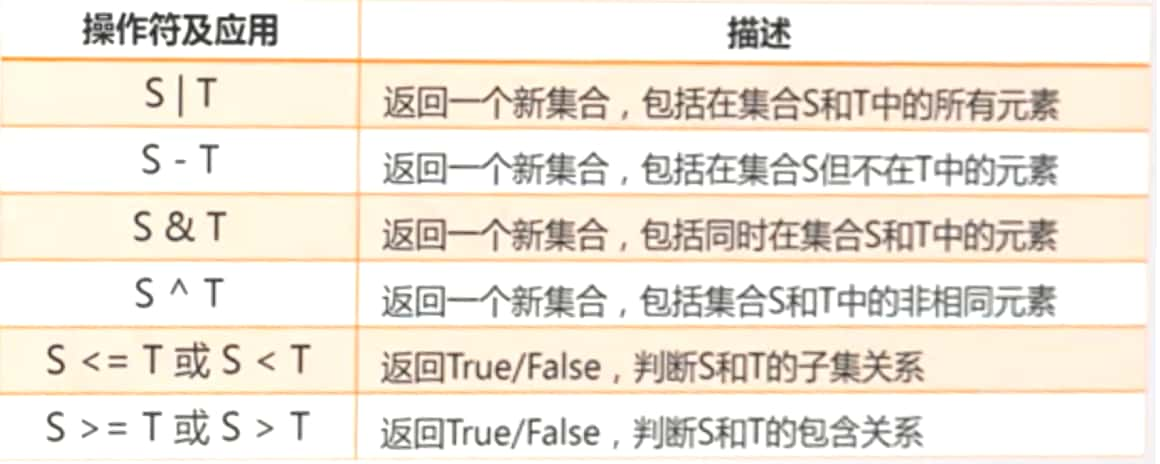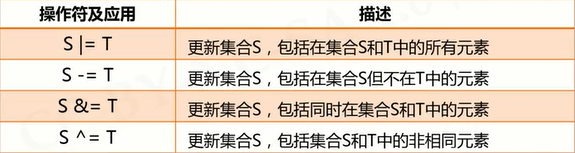>>> A = {
"p", "y", 123}
>>> B = set("pypy123")
>>> A - B
{
123}
>>> B - A
{
'2', '3', '1'}
>>> A & B
{
'y', 'p'}
>>> A | B
{
'y', 'p', '3', '2', 123, '1'}
>>> A ^ B
{
'3', '2', 123, '1'}


S.reamove(x)

S.clear()

S.pop()

S.copy()

len(S)

set(x)

>>> ls = ["p", "p", "y", "y", 123]
>>> s = set(ls)
>>> lt = list(s)
>>> print(lt)
[123, 'y', 'p']


##### 序列类型

len(s)

>>> ls = ["python", 123, ".io"]
>>> len(ls)
3


min(s)

max(s)

>>> s = "python123.io"
>>> max(s)
'y'


s.index(x) or s.index(x,i,j)

s.count(x)

##### 元组类型

>>> a = 1,2
>>> a
(1, 2)

>>> b = (1,2)
>>> b
(1, 2)


##### 列表类型

del ls[i]

del ls[i:j:k]

ls += lt

###### ls *= n

>>> ls = ["cat", "dog", "tiger", 1024]
>>> ls[1:2] = [1,2,3,4]
>>> print(ls)
['cat', 1, 2, 3, 4, 'tiger', 1024]
>>> del ls[::3]
>>> print(ls)
[1, 2, 4, 'tiger']
>>> ls *2
[1, 2, 4, 'tiger', 1, 2, 4, 'tiger']


ls.append(x)

ls.clear()

ls.copy()

ls.insert(i,x)

ls.pop(i)

ls.remove(x)

ls.reverse()

##### 字典类型

>>> d = {
"中国":"北京", "美国":"华盛顿", "法国":"巴黎"}
>>> d
{
"中国":"北京", "美国":"华盛顿", "法国":"巴黎"}
>>> d["中国"]
'北京'


del d[k]

d.keys()

d.values()

d.items()

k in d

>>> d = {
"中国":"北京", "美国":"华盛顿", "法国":"巴黎"}
>>> "中国" in d
True

>>> d.keys()
dict_keys(['中国', '美国', '法国'])

>>> d.values()
dict_values(['北京', '华盛顿', '巴黎'])


d.get(k, <>)

d.pop(k, <>)

d.popitem()

d.clear()

len(d)

>>> d = {
"中国":"北京", "美国":"华盛顿", "法国":"巴黎"}
>>> d.get("中国", "伊斯兰堡")
'北京'

>>> d.get("巴基斯坦", "伊斯兰堡")
'伊斯兰堡'

>>> d.popitem()
('美国', '华盛顿')


### jieba库

jieba.lcut(s)

>>> jieba.lcut("中国是一个伟大的国家")
['中国', '是', '一个', '伟大', '的', '国家']


>>> jieba.add_word("呵呵哒")


### wordcloud库

wordcloud库把词云当作一个WordCloud对象

• wordcloud.WordCloud()代表一个文本对应的词云
• 可以根据文本中词语出现的频率等参数绘制词云
• 绘制词云的形状、尺寸、颜色都可以设定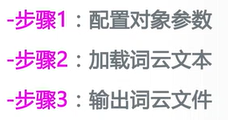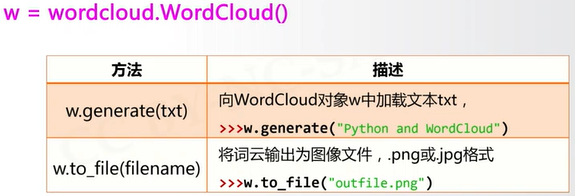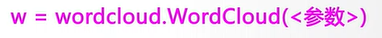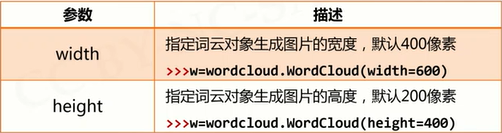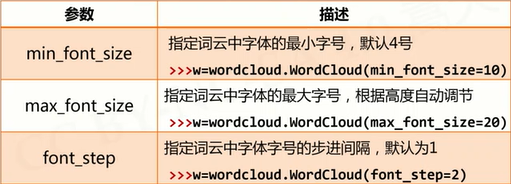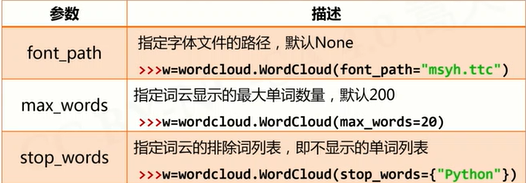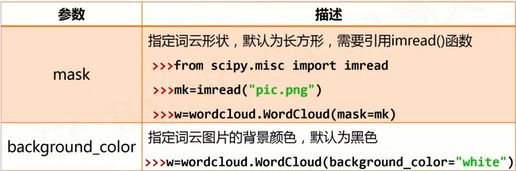### 坑：

# 生成英文词云
import wordcloud
txt  = "life is short, you need python"
w = wordcloud.WordCloud(background_color = 'white')
w.generate(txt)
w.to_file("pywcloud.png")

# 生成中文词云（中文需要先分词）
import jieba
import wordcloud
txt  = "程序设计语言是计算机能够理解和识别用户操作意图的一种交互体系，\
它按照特定规则组织计算机指令，\
使计算机能够自动进行各种运算处理。"
w = wordcloud.WordCloud(width=1000, font_path="msyh.ttc", height=700)
w.generate(" ".join(jieba.lcut(txt)))
w.to_file("pywcloud.png")


### 文件

##### 文件的打开关闭

<变量名> = open(<文件名>, <打开模式>)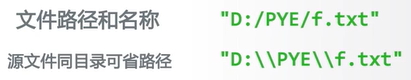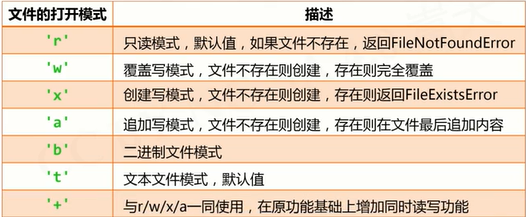<变量名>.close()

##### 文件内容的读取

>>> s = f.read(2)



fname = input("请输入要打开的文件名称：")
fo = open(fname, "r")
while txt !=- "" :
# 对txt进行处理
fo.close()


>>> s = f.readline()



>>> s = f.readlines()
[‘中国是一个伟大的国家！’]

# 逐行遍历文件：方法一
fname = input("请输入要打开的文件名称：")
fo = open(fname, "r")
print(line)
fo.close()

# 逐行遍历文件：方法二
fname = input("请输入要打开的文件名称：")
fo = open(fname, "r")
for line in fo:
print(line)
fo.close()


##### 数据文件的写入

f.write(s)

>>> f.write("中国是一个伟大的国家！")


f.writelines(lines)

>>> ls = ["中国", "法国", "美国"]
>>> f.writelines(ls) # 没有换行、空格等，直接连接


fo = open("output.txt", "w+")
ls = ["中国", "法国", "美国"]
fo.writelines(ls)
fo.seek(0) # 如果不将光标回到文件开始，将不会有输出结果
for line in fo:
print(line)
fo.close()


f.seek(offset)

0 - 文件开头；1 - 当前位置；2 - 文件结尾

>>> f.seek(0) # 回到文件开头


##### 一维数据的读、写处理

txt = open(fname).read()
ls = txt.split()
f.close()


ls = ['中国', '美国', '日本']
f = open(fname, 'w')
f.write(' '.join(ls)) # 将空格加入到ls各元素之间
f.close()


##### 二维数据的读、写处理

fo = open(fname)
ls = []
for line in fo:
line = line.replace("\n","") # 去除每行最后的换行符
ls.append(line.split(","))
fo.close()


ls = [[], [], []] # 二维列表
f = open(fname, 'w')
for item in ls:
f.write(','.join(item) + '\n')
f.close()


### OS库

os库是提供通用的、基本的操作系统交互功能的标准库

• 路径操作：os.path子库，处理文件路径及信息
• 进程管理：启动系统中其他程序
• 环境参数：获得系统软硬件信息等环境参数

##### 路径操作

os.path子库以path为入口，用于操作和处理文件路径

import os.path
import os.path as op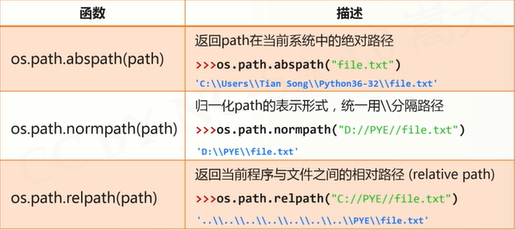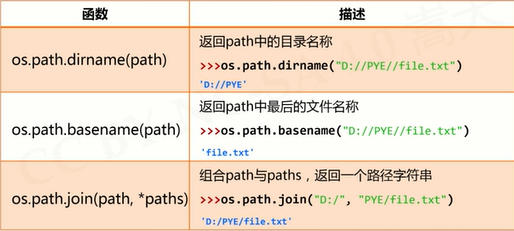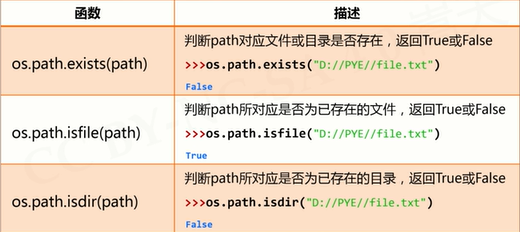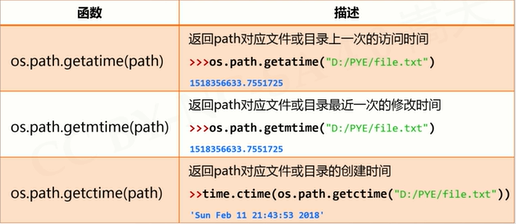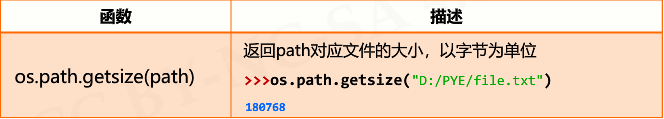##### 进程管理

os.system(command)

• 执行程序或命令command
• 在Windows系统中，返回值为cmd的调用返回信息
>>> import os
>>> os.system("C:\\Windows\\System32\\calc.exe") # 调用计算器程序
0

>>> import os
>>> os.system("C:\\Windows\\System32\\calc.exe \
D:\\PYECourse\\grwordcloud.png")
# 调用画图程序打开文件（路径间用空格隔开）
0

# 批量安装第三方库
import os
libs = {
"numpy", "sklearn", "pandas", "jieba"}

try:
for lib in libs:
os.system("pip install " + lib)
print("Successful")
except:
print("Failed Somehow")


##### 环境参数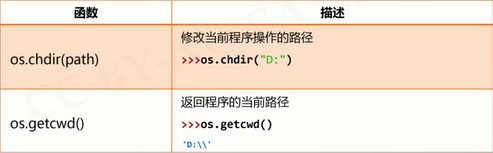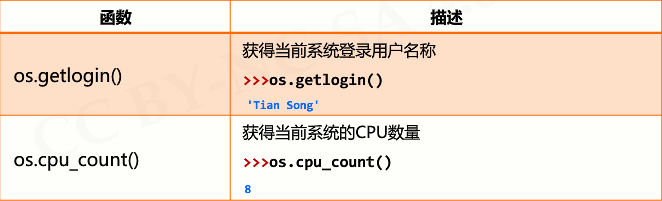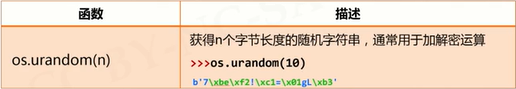### 一些NB的库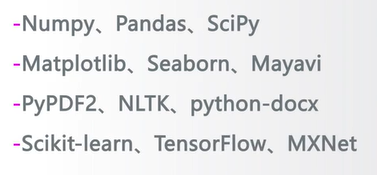### python pip命令报错 invlid systax_pip执行命令过程报错-SyntaxError: invalid syntax_weixin_39841825的博客-程序员宅基地

pip执行命令过程中出现错误，异常代码如下：Traceback (most recent call last):File "/usr/bin/pip", line 7, in from pip._internal.main import mainFile "/usr/lib/python2.6/site-packages/pip/_internal/main.py", line 13, in fr...

### 中国情侣必去的国内十大浪漫圣地_ximenchuixuezijin的博客-程序员宅基地

1、丽江——追寻一米阳光的传说         清冷的玉龙雪山顶上，终年云雾缭绕，即使是在最晴朗的天气，阳光也很难穿透云层，传说每年秋分是日月交合同辉同映的日子，只有在特别偶然的时刻，才能看到有一米长的阳光照在山顶，而被这道阳光照耀到的人就能拥有一世不变的爱情。也许，这一瞬间

### jdbc连接池java_JDBC连接池-自定义连接池_梧桐应恨夜来霜的博客-程序员宅基地

JDBC连接池java JDBC连接中用到Connection 在每次对数据进行增删查改 都要 开启 、关闭 ，在实例开发项目中 ，浪费了很大的资源 ，以下是之前连接JDBC的案例packagecom.jdbc.connection;importjava.io.IOException;importjava.io.InputStream;importjava.sql.Connection;i...

### orcale的DDL与DML_☆枭★的博客-程序员宅基地

sql语句类型：DDL:数据库定义语言DML:数据库操作语言 增删改DCL:数据控制语言DML：增加：insert into 表名（字段名） values （字段值）；删除：delect from 表名 where 字段改：update 表名 set 字段=‘值’ where 字段插入：&amp; 动态插入insert into emp（ename） values （&amp;e...

### vue 目录名称详解_vue目录详解_weixin_39673471的博客-程序员宅基地

1. build文件夹：打包配置的文件夹2. config文件夹：打包的配置，webpack对应的配置3. src文件夹：开发项目的源码4. App.vue ： 入口组件 :项目入口文件，页面级vue组件5. static文件夹：静态资源，图片6. .babelrc：ES6解析的配置7. .gitignore：忽略某个或一组文件git提交的一个配置8. index.html：单页面的入口，通过we...